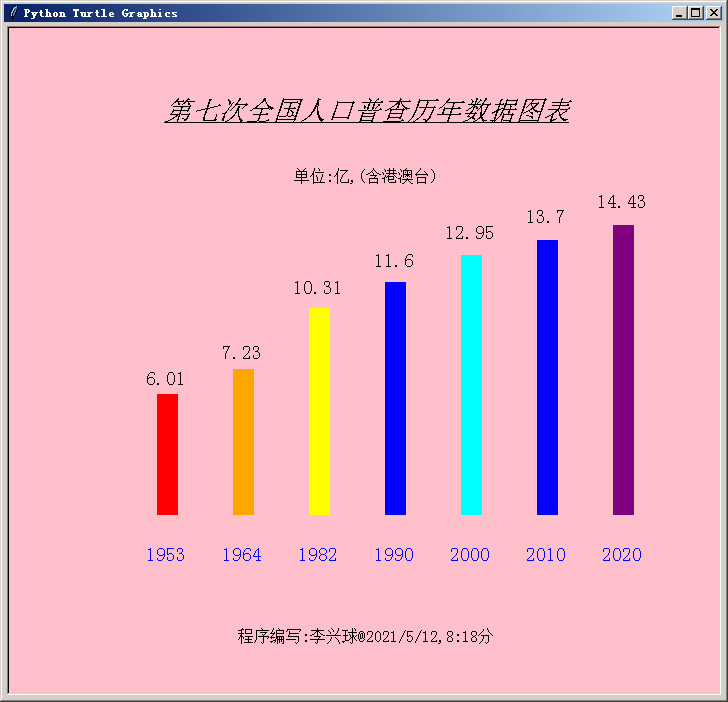# Python海龟数据分析，第七次全国人口普查历年数据图表.py## 如本文章标有价格,需议价或其它事情商议请加微信pythonxia李兴球Python第七次全国人口普查历年数据图表
```"""
Python海龟数据分析，第七次全国人口普查历年数据图表.py
使用Python的海龟模块能进行数据分析，显示统计图标，画柱状图吗?
答案是肯定的。下面的程序主要使用了变形shapesize和图章功能。
把每一年人口数据以柱状图的形式显示出来。

"""
import turtle

years = [1953,1964,1982,1990,2000,2010,2020]      # 年份表
datas = [6.01,7.23,10.31,11.60,12.95,13.70,14.43] # 数据含港澳台，单位:亿
cs = ['red','orange','yellow','blue','cyan','blue','purple'] # 颜色表

points =((0,0),(0,10),(-20,10),(-20,-10),(0,-10))
turtle.addshape('csquare',points)
turtle.shape('csquare')

turtle.bgcolor("pink")               # 背景色为粉红色
turtle.penup()                       # 抬起笔来

turtle.goto(-200,-150)               # 定位到此开始盖图章
turtle.hideturtle()

ft = ('',14,'normal')
for i in range(7):
c = cs[i]
year = years[i]
人口数量 = datas[i]              # 使用中文更好理解
turtle.color(c)
turtle.shapesize(人口数量,1)     # 变形
turtle.stamp()                   # 盖图章(柱状图)
# 下面这4行代码是写年份，需要先调整y坐标
turtle.color('blue')
turtle.sety(turtle.ycor()-50)
turtle.write(year,align='center',font=ft)
turtle.sety(turtle.ycor()+50)
# 下面这4行代码是写人口数量。
turtle.color('black')
turtle.sety(turtle.ycor()+人口数量*21)
turtle.write(人口数量,align='center',font=ft)
turtle.sety(turtle.ycor()-人口数量*21)

turtle.setx(turtle.xcor()+76)    # 海龟往右移76个单位

turtle.goto(0,240)
ft = ('',20,'underline italic')
s = '第七次全国人口普查历年数据图表'
turtle.write(s,align='center',font=ft)

turtle.goto(0,180)
ft = ('',12,'normal')
s = '单位:亿,(含港澳台)'
turtle.write(s,align='center',font=ft)

turtle.goto(0,-280)
ft = ('',12,'normal')
s = '程序编写:李兴球@2021/5/12,8:18分'
turtle.write(s,align='center',font=ft)

```scratch